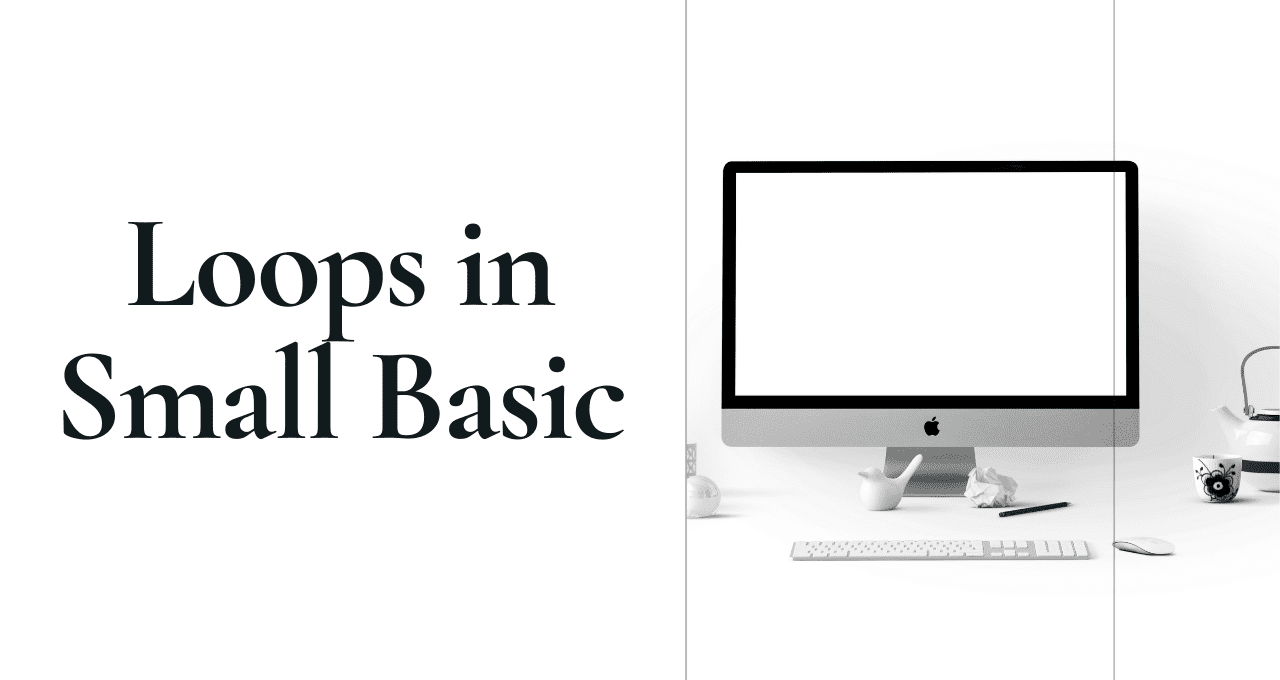In this article, you will get Important Small Basic Loops Class7. So here we start!

Topics Covered

## Important QnA Small Basic Loops Class7

In the starting of MS Small Basic Looping Statements Class 7 I will provide you answers of Fill in the blanks, True/False, and MCQs.

## Fill in the blanks

1. For..EndFor
2. Graphics Window
3. Step
4. Black
5. DrawRectangle

## True/Flase

1. True
2. False – Correction:The for loop is executed when the condition is True.
3. False – Correction:The default value of the step clause is 1 in the for statement.
4. True
5. True

## MCQs

1. iv. 20 16 12
2. ii. 3
3. iv. GraphicsWindow.Fillrectangle(100,100,100,100)
4. iv. GraphicsWindow.Height=400
5. iv. Both i and iii

In the next section of Qna Small Basic Loops Class7, we will see subjective type questions and answers:

1. Write the looping statements available in small basic.

Ans. – There are two types of looping statements available in small basic.

1. For…EndFor
2. While…EndWhile

2. What are the parts of a loop? Explain with example.

Ans. There are three parts of loop:

1. Iterator – The starting point
2. Condition – To limit the loop or end point of the series
3. Update Statement – Change the value after execution of the statement

3. Write code to print a series starts with 23 and ends with 70 using while loop.

``````i = 23
while i<=70
TextWindow.WriteLine(i)
i = i + 1
EndWhile``````

4. Write above code using for loop.

``````For i=23 to 70 Step 1
TextWindow.WriteLine(i)
EndFor``````

5. In which situation you will use the for loop?

Ans. The for loop is used when the repetition of statements block are known to the programmer.

6. What do you mean step in for loop?

Ans. The step in for loop plays an important role to update the value of iterator. If it is not specified Small Basic will consider step value as 1.

7. Write code display the series in reverse order using while loop. Ask user to enter start value and stop value.

``````TextWindow.Write("Enter the start value(Max. Value):")
TextWindow.Write("Enter the end value(Least Value):")
While i>=ev
TextWindow.WriteLine(i)
i = i - 1
EndWhile``````

8. Write above code using for loop.

``````TextWindow.Write("Enter the start value(Max. Value):")
TextWindow.Write("Enter the end value(Least Value):")
For i=sv to ev Step -1
TextWindow.WriteLine(i)
EndWhile``````

9. What do you mean by endless loop?

Ans. A loop which doesn’t contain any statement that is going to evaluate false is known as endless loop.

In the next section of small basic loops class7, we will cover questions related to Graphics.

10. What is the significance of GraphicsWindow in small basic?

Ans. The GraphicsWindow is one of the object of MS Small Basic which is used to draw lines, shapes, and text with various colors.

11. How to change the size of window in MS Small Basic?

Ans. To change the size of window in MS Small Basic use the appropriate value of X and Y coordinates. These X and Y are the pixel values which changes the vertical and horizontal co-ordinates of a window. So here in Small Basic you can use Height and Width properties of window to change the size. For Example,

``````
GraphicsWindow.Height = 200
GraphicsWindow.Width = 200``````

The default value of Height is 444 and default value of width is 624 in MS Small Basic.

12. Write common properties of GraphicsWindow of MS Small Basic.

13. Write the font properties of GraphicsWindow of MS Small Basic.

14. What are the methods of GraphicsWindow?

Now in the next section of small basic loops class7, we will some application based questions

1. Write the output of the following code:

Code 1:

``````i = 10
While i<15
TextWindow.Write(i + " ")
i = i + 2
EndWhile``````

Code 2:

``````For i=45 to 30 Step -5
TextWindow.Write(i + " ")
EndFor``````

Code 3:

``````For i=10 to 6 Step -2
TextWindow.Write(i*2 + " ")
EndFor``````

2. How many times following loop will be executed?

Code 1:

``````i = 7
While i<=35
TextWindow.Write(i)
i = i + 7
EndWhile``````

Code 2:

``````For i=12 to 45 Step 3
TextWindow.Write(i)
i=i+3
EndFor``````

Dear Students, That’s all from this chapter small basic loops class7. If you have any doubt regarding small basic loops class7, you ask in comment. Don’t forget to share your views in the comment section.

If you missed any chapter, links are provided below:

Chapter 1 Writing formulas in MS Excel

Chapter 2 Creating Charts in MS Excel

Chapter 3 Computer Languages and Number System

##### 2 thoughts on “Important QnA Small Basic Loops Class7”
1.Saatvik Joshi says:

2.Aniruddha says: